# JP4019813B2 - Physical quantity estimation device, road friction state estimation device, steering angle neutral point estimation device, and air pressure drop estimation device - Google Patents

Physical quantity estimation device, road friction state estimation device, steering angle neutral point estimation device, and air pressure drop estimation device Download PDF

## Info

Publication number
JP4019813B2
JP4019813B2 JP2002183413A JP2002183413A JP4019813B2 JP 4019813 B2 JP4019813 B2 JP 4019813B2 JP 2002183413 A JP2002183413 A JP 2002183413A JP 2002183413 A JP2002183413 A JP 2002183413A JP 4019813 B2 JP4019813 B2 JP 4019813B2
Authority
JP
Japan
Prior art keywords
steering
self
value
aligning torque
sat
Prior art date
Legal status (The legal status is an assumption and is not a legal conclusion. Google has not performed a legal analysis and makes no representation as to the accuracy of the status listed.)
Expired - Fee Related
Application number
JP2002183413A
Other languages
Japanese (ja)
Other versions
JP2003127888A (en
Inventor

Original Assignee

Priority date (The priority date is an assumption and is not a legal conclusion. Google has not performed a legal analysis and makes no representation as to the accuracy of the date listed.)
Filing date
Publication date
Priority to JP2001212683 priority Critical
Priority to JP2001-212683 priority
Application filed by 株式会社豊田中央研究所 filed Critical 株式会社豊田中央研究所
Priority to JP2002183413A priority patent/JP4019813B2/en
Publication of JP2003127888A publication Critical patent/JP2003127888A/en
Application granted granted Critical
Publication of JP4019813B2 publication Critical patent/JP4019813B2/en
Application status is Expired - Fee Related legal-status Critical
Anticipated expiration legal-status Critical

## Images

•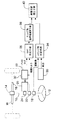•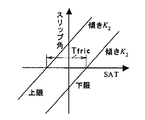•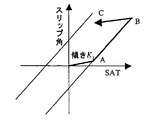••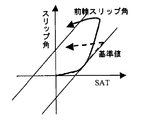•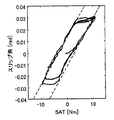•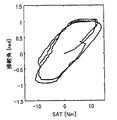•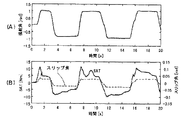•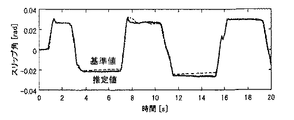••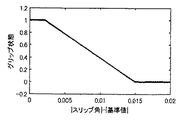•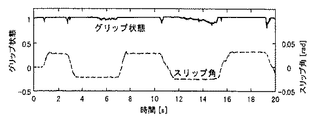•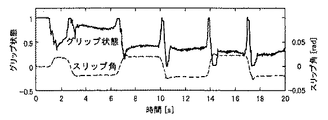•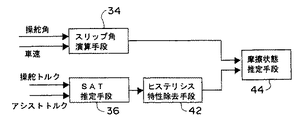•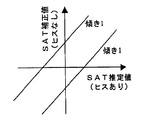•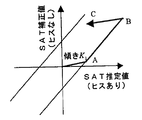•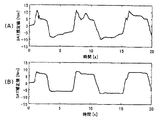•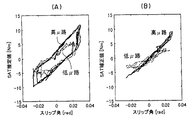•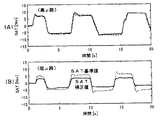•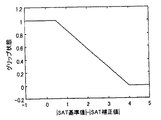••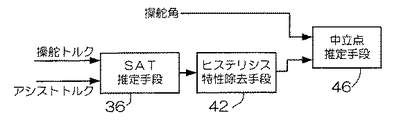•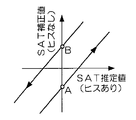•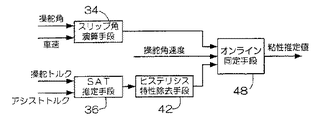•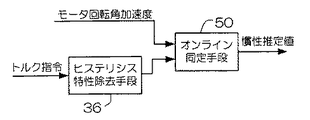•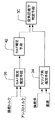•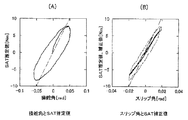••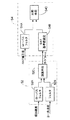•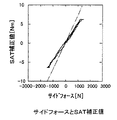## Classifications

• GPHYSICS
• G01MEASURING; TESTING
• G01NINVESTIGATING OR ANALYSING MATERIALS BY DETERMINING THEIR CHEMICAL OR PHYSICAL PROPERTIES
• G01N19/00Investigating materials by mechanical methods
• G01N19/02Measuring coefficient of friction between materials
• BPERFORMING OPERATIONS; TRANSPORTING
• B62LAND VEHICLES FOR TRAVELLING OTHERWISE THAN ON RAILS
• B62DMOTOR VEHICLES; TRAILERS
• B62D15/00Steering not otherwise provided for
• B62D15/02Steering position indicators ; Steering position determination; Steering aids
• BPERFORMING OPERATIONS; TRANSPORTING
• B60VEHICLES IN GENERAL
• B60TVEHICLE BRAKE CONTROL SYSTEMS OR PARTS THEREOF; BRAKE CONTROL SYSTEMS OR PARTS THEREOF, IN GENERAL; ARRANGEMENT OF BRAKING ELEMENTS ON VEHICLES IN GENERAL; PORTABLE DEVICES FOR PREVENTING UNWANTED MOVEMENT OF VEHICLES; VEHICLE MODIFICATIONS TO FACILITATE COOLING OF BRAKES
• B60T2210/00Detection or estimation of road or environment conditions; Detection or estimation of road shapes
• B60T2210/10Detection or estimation of road conditions
• B60T2210/12Friction

## Description


BACKGROUND OF THE INVENTION
The present invention relates to a physical quantity estimation device, a road surface friction state estimation device, a steering angle neutral point estimation device, and a tire air pressure drop estimation device, and in particular, a physical quantity estimation device that estimates a physical quantity based on a correction value from which hysteresis characteristics are removed, A road friction state estimation device that obtains a correction value by removing hysteresis characteristics caused by the influence of viscous friction such as coulomb friction from the estimated value of self-aligning torque (SAT), and estimates the road surface friction state based on the obtained correction value Steering angle neutral point estimation device for estimating neutral point of steering angle based on correction value and steering angle from which hysteresis characteristic has been removed, and decrease in tire air pressure based on correction value and SAT reference value from which hysteresis characteristic has been removed The present invention relates to a tire pressure drop estimation device for estimating the tire pressure.

[Prior art and problems to be solved by the invention]
Japanese Patent Application Laid-Open No. 11-287749 detects the steering angle and steering torque of a tire, calculates the characteristics of the steering torque with respect to the steering angle, and based on the calculation result, the friction coefficient μ (road surface) A technique for computing μ) is disclosed.

In this prior art, since the road surface μ, which is a physical quantity corresponding to the grip state, is obtained as the amount of change in the steering torque with respect to the amount of change in the steering angle, it is an estimation method that is easily affected by noise. In other words, obtaining the amount of change means performing differentiation to amplify the noise, and therefore the estimated value is a value containing a lot of noise.

Further, in this prior art, since the road surface μ is estimated only when the steering angle is increased, the friction coefficient cannot be estimated because the increase cannot be performed at the maximum steering angle at which the load applied to the tire is the largest. Originally, the estimation accuracy is improved by estimating the road surface μ when the load applied to the tire is the largest, that is, near the limit, that is, estimating the road surface μ of the limit. There is a problem in that μ cannot be estimated and the road surface μ can be estimated only before reaching the maximum steering angle.

By the way, a hysteresis characteristic is generated between the SAT and the slip angle or the steering angle due to the twist of the tire tread, the Coulomb friction of the power steering device, or the like. For this reason, the characteristics differ between when the steering angle is increased and when it is switched back, and the estimated value varies in the conventional method that focuses on the change in the SAT with respect to the change in the slip angle or the steering angle.

Also, if the driver decreases the steering force to such an extent that the steering wheel does not move during steering, the SAT decreases despite the fact that the slip angle or the steering angle does not change, resulting in a “grip state decreased” There is a possibility of misjudgment. That is, in the above-described prior art, estimation is performed only at the time of additional cutting to avoid such a misjudgment and to reduce the variation of the estimated value. There is a problem that cannot be estimated.

In addition, the fact that the road surface friction state cannot be estimated at the time of switching back and steering means that the grip state changes, for example, when switching from a low μ road to a high μ road in a steering state or from a high μ road to a low μ road. In this case, the road surface friction state cannot be estimated at the time of change, and the grip state cannot be estimated until the steering angle is increased. For this reason, the estimated value of the grip state according to the prior art cannot be used as a control parameter for switching the characteristics of the power steering apparatus or ABS that requires fast adaptability according to the grip state.

In Japanese Patent Laid-Open Nos. 11-334634 and 11-59466, a reference steering torque set based on the steering angle and the vehicle speed is compared with the steering torque, and the steering torque is larger than the reference steering torque. Describes a technique for determining that the tire air pressure has decreased when the air pressure continues for a certain period of time.

In the above prior art, since the steering torque including the friction of the steering system is used, the change of the steering torque with respect to the air pressure is affected by this friction, so that it cannot be accurately detected, and the air pressure is highly accurate due to the influence of the friction. There is a problem that it is not possible to estimate the decrease in.

Further, the steering angle and the steering torque are affected by the dynamic characteristics of the vehicle motion in addition to the friction of the steering system. For this reason, there is a concern that the estimation accuracy may deteriorate when fast steering is performed.

As a countermeasure against the above problem, the prior art adds a condition that the state where the steering torque is larger than the reference steering torque continues for a certain period of time, and this condition alleviates the problem of accuracy degradation. However, there is a new problem that the estimation opportunities are reduced by this condition, and as a result, the estimation time is delayed.

The present invention has been made in order to solve the above problems, and in order to prevent variations in estimated values, a physical quantity estimation device capable of estimating a physical quantity with high accuracy by removing hysteresis characteristics, and a steering angle are provided. A road surface friction state estimation device that can accurately estimate the road surface friction state without increasing the angle, a steering angle neutral point estimation device that can accurately estimate the neutral point of the steering angle, and a tire pressure drop estimation An object of the present invention is to provide a tire pressure drop estimation device that can be used.

[Means for Solving the Problems]
In order to achieve the above object, the physical quantity estimation apparatus of the present invention provides: For steering wheel angle or steering wheel slip angle A first output means for outputting a steering state of a steered wheel having no hysteresis characteristic; a second output means for outputting a self-aligning torque estimated value estimated from a steering torque and an assist torque; and the self-aligning. From estimated torque , Against steering wheel angle or steering wheel slip angle Hysteresis removing means for removing a hysteresis characteristic and calculating a self-aligning torque correction value, and one of a wheel state and a road surface state during traveling of the vehicle based on the steering state of the steering wheel and the self-aligning torque correction value And an estimation means for estimating a physical quantity.

According to the present invention, For steering wheel angle or steering wheel slip angle From estimated self-aligning torque with hysteresis characteristics For steering wheel angle or steering wheel slip angle Since the physical quantity related to one of the wheel state and the road surface condition during traveling of the vehicle is estimated based on the self-aligning torque correction value from which the hysteresis characteristic has been removed, the physical quantity with high accuracy can be estimated. As a result, a physical quantity related to one of the wheel state and road surface condition during traveling of another vehicle can be estimated from a highly accurate self-aligning torque correction value, so that the estimated physical quantity does not vary.

In the present invention, the steering state of the steered wheel is, for example, Handle angle or The slip angle of the steering wheel can be set.

This self-aligning torque correction value is an arithmetic expression having a gradient represented by the ratio of the change of the self-aligning torque correction value to the change of the self-aligning torque estimated value, and the self-aligning torque estimated value by Coulomb friction It is possible to calculate with a different calculation formula for each region in which the gradient of the region where the fluctuation is smaller than the gradient of the region other than the region.

In addition, the hysteresis characteristic represents a predetermined physical relationship between a physical quantity having a hysteresis characteristic and the same physical quantity having no hysteresis characteristic as a graph or a table, and has a hysteresis characteristic based on this physical relation. It can be removed by converting the physical quantity into a physical quantity having no hysteresis characteristic. In this case, conversion is facilitated by predetermining the initial value according to the previous history.

A physical quantity (for example, a decrease in tire air pressure or a road surface friction state) related to one of a wheel state and a road surface state when the vehicle travels is determined by the slip of the steering wheel. Horns and Based on the self-aligning torque correction value or slip of the steered wheels In the corner It can be estimated based on the self-aligning torque reference value and the self-aligning torque correction value set accordingly.

Also, the slope of the self-aligning torque correction value expressed as the ratio of the change in the self-aligning torque correction value to the change in the slip angle. To Based on this, it is possible to estimate a physical quantity related to one of the wheel state and the road surface state during vehicle travel.

Further, the physical quantity related to one of the wheel state and the road surface state during vehicle travel can be estimated based on the slip angle of the steered wheel and either the slip angle reference value or the self-aligning torque correction value.

The slip angle reference value is an arithmetic expression having a gradient represented by the ratio of the change in the slip angle reference value to the change in the self-aligning torque estimated value, and is a region where the self-aligning torque estimated value varies due to Coulomb friction. The gradient may be calculated by a different calculation formula for each region in which the gradient is smaller than the gradient of the region other than the region.

A road surface friction state estimating device of the present invention includes a steering angle sensor that detects a steering angle, a vehicle speed sensor that detects a vehicle speed, a torque sensor that detects steering torque, an assist torque sensor that detects steering assist torque, and a steering A slip angle estimating means for estimating a slip angle of a steered wheel based on an angle and a vehicle speed; a self aligning torque estimating means for outputting a self aligning torque estimated value based on a steering torque and an assist torque; Based on the relationship between the self-aligning torque estimated value and the slip angle, for the self-aligning torque estimated value, The hysteresis characteristic for the steering wheel angle or the steering wheel slip angle has been removed. It includes hysteresis removing means for calculating a slip angle reference value, and friction state estimating means for estimating a road surface friction state from the slip angle reference value and the slip angle.

The slip angle estimating means of the road surface friction state estimating device of the present invention estimates the slip angle of the steered wheel (for example, the front wheel) using the steering angle and the vehicle speed based on the vehicle motion model. The self-aligning torque (SAT) estimating means estimates the SAT that is the road surface reaction force by adding the steering torque and the assist torque, specifically, based on the steering torque and the assist torque. The steering torque is detected by, for example, a torque sensor mounted coaxially with the steering wheel, and the assist torque is calculated from the current of the electric power steering device, for example.

The SAT estimation accuracy can be improved by subtracting the viscous friction torque generated in proportion to the steering angular velocity from the value obtained by adding the steering torque and the assist torque. Further, the SAT may be estimated by the disturbance observer method described in Japanese Patent Application No. 2000-370704 using the steering angular velocity in addition to the steering angle. By using the disturbance observer method, it is possible to estimate the SAT in consideration of the torque generated by the inertia of the power steering apparatus.

The hysteresis removing means is based on the relationship between the self-aligning torque estimated value and the slip angle. For steering wheel angle or steering wheel slip angle A slip angle reference value for the estimated self-aligning torque value from which the hysteresis characteristic has been removed is calculated.

That is, the hysteresis removing means can calculate the slip angle with respect to the self-aligning torque estimated value as a correction value based on the physical relationship between the self-aligning torque having hysteresis characteristics and the slip angle. That is, a slip angle (slip angle reference value) that takes into account hysteresis characteristics caused by Coulomb friction of the power steering device or the like from the SAT estimated value estimated by the SAT estimating means is output as a correction value. Since the slip angle reference value is a slip angle of a steered wheel, for example, a front wheel, generated in a steering region where the grip state is sufficiently high and there is a margin, the hysteresis characteristic with respect to the estimated value of the self-aligning torque is removed.

The friction state estimating means compares the slip angle of the steered wheel estimated by the slip angle estimating means with the slip angle reference value output from the hysteresis removing means, and the slip angle is reduced as the grip state, that is, the road surface friction state is lowered. The grip state, that is, the road surface friction state is estimated by utilizing the fact that the slip angle becomes larger than the reference value.

Further, the hysteresis removing means replaces the above-described removal method with a hysteresis from the self-aligning torque estimated value based on a physical relationship between the self-aligning torque estimated value and the self-aligning torque correction value from which the hysteresis characteristic is removed. The self-aligning torque from which the characteristics are removed may be calculated as a self-aligning torque correction value. In this case, a self-aligning torque (SAT correction value) obtained by removing the hysteresis characteristic directly from the estimated value of the self-aligning torque is calculated.

The friction state estimating means compares the SAT reference value calculated by multiplying the slip angle of the steered wheel estimated by the slip angle estimating means with a coefficient that changes according to the vehicle speed and the tire type, and the SAT correction value. The grip state, that is, the road surface friction state is estimated by utilizing the fact that the SAT correction value becomes smaller than the SAT reference value as the state, that is, the road surface friction state decreases.

As described above, according to the present invention, the correction value from which the hysteresis characteristic is removed is output based on the self-aligning torque, and the road surface friction state is estimated based on this correction value. The road surface friction state can be estimated accurately at

The steering angle neutral point estimation device of the present invention includes a steering angle sensor that detects a steering angle, a torque sensor that detects a steering torque, an assist torque sensor that detects steering assist torque, a steering torque, and an assist torque. A self-aligning torque estimating means for outputting a self-aligning torque estimated value based on the self-aligning torque estimated value, Remove the hysteresis characteristics for the steering wheel angle or the slip angle of the steering wheel, Based on the hysteresis removing means for calculating the self-aligning torque correction value using the value when the estimated value becomes 0 as the initial value, and the self-aligning torque correction value and the steering angle, Neutral point estimating means for estimating the steering angle when the value becomes 0 as the neutral point of the steering angle.

The self-aligning torque (SAT) estimating means of the present invention estimates the SAT based on the steering torque and the assist torque as described above. The hysteresis removing means uses the value when the estimated value of SAT becomes 0 as the initial value, For steering wheel angle or steering wheel slip angle SAT having hysteresis characteristics For steering wheel angle or steering wheel slip angle From the physical relationship with SAT without the hysteresis characteristics For steering wheel angle or steering wheel slip angle The SAT correction value from which the hysteresis characteristic has been removed is output. Then, based on the SAT correction value and the steering angle, the neutral point estimation means estimates the steering angle when the correction value becomes 0 as the neutral point of the steering angle.

A tire pressure drop estimation device according to the present invention includes a lateral acceleration sensor that detects lateral acceleration, a yaw angular velocity sensor that detects a yaw angular velocity, a torque sensor that detects steering torque, and an assist torque sensor that detects steering assist torque. And a side force estimating means for estimating the side force of the steered wheel based on the lateral acceleration and the yaw angular velocity, and a self-aligning torque estimation for outputting a self-aligning torque estimated value based on the steering torque and the assist torque. Means and the self-aligning torque estimate For steering wheel angle or steering wheel slip angle Hysteresis removal means for removing a hysteresis characteristic and calculating a self-aligning torque correction value, and an air pressure decrease for estimating whether the tire air pressure has decreased based on the self-aligning torque correction value and the side force And an estimation means.

In this tire pressure drop estimation device, instead of the steering angle sensor and the vehicle speed sensor, a lateral acceleration sensor for detecting lateral acceleration and a yaw angular velocity sensor for detecting yaw angular velocity are provided, and the lateral acceleration and the yaw angular velocity are converted to each other. The side force of the steered wheel may be estimated based on the self-aligning torque correction value and the side force, and it may be estimated whether or not the tire air pressure has decreased.

The decrease in tire air pressure is based on the self-aligning torque correction value and the self-aligning torque reference value set according to the steering wheel slip angle, and the self-aligning torque correction value and the side force of the steering wheel. On the basis of the self-aligning torque reference value set in accordance with or based on the gradient of the self-aligning torque correction value represented by the ratio of the change in the self-aligning torque correction value to the change in the slip angle, Can be estimated.

Further, based on the gradient of the self-aligning torque correction value represented by the ratio of the change in the self-aligning torque correction value to the change in the side force, it may be estimated whether or not the tire air pressure has decreased. .

The ratio between the SAT correction value and the SAT reference value calculated by the air pressure decrease determination means is a value that increases when the air pressure decreases, and when this value exceeds a threshold value, it is possible to determine the air pressure decrease. However, this value is also a value representing the grip state at the same time, and becomes a small value when the grip is lowered, such as when traveling at a low μ. For this reason, if the ratio between the SAT correction value and the SAT reference value exceeds the threshold value for determining the air pressure, it is determined that the air pressure has decreased, and the ratio between the SAT correction value and the SAT reference value falls below the threshold value for determining the grip decrease. In this case, it can be determined that the grip is lowered.

Note that a decrease in the ratio between the SAT correction value and the SAT reference value due to the grip reduction occurs with steering, and the change is relatively fast, whereas the SAT correction value and the SAT reference due to the decrease in air pressure. The increase in ratio with the value is slowly changing. For this reason, when the ratio between the SAT correction value and the SAT quasi-value is identified by the online least square method, the air pressure estimation logic sets a larger forgetting factor or decimation than the grip degree estimation logic. Therefore, it is desirable to capture a slow change in the ratio between the SAT correction value and the SAT reference value by thinning out the data. In addition, when determining the decrease in air pressure, it is desirable to use data in the vicinity of a straight traveling state with a small slip angle that is not affected by a decrease in grip, so data when steering is performed with a relatively large slip angle. Can be removed by sorting.

DETAILED DESCRIPTION OF THE INVENTION
DESCRIPTION OF THE PREFERRED EMBODIMENTS Embodiments in the case where the present invention is applied to a vehicle that is steered by front wheels will be described below in detail with reference to the drawings. First, the road surface friction state estimation apparatus according to the first embodiment will be described.

As shown in FIG. 1, an electric power steering apparatus mounted on a vehicle is provided with a steering wheel (handle) 12 fixed to an upper end portion of a steering shaft 10. A lower end portion of the steering shaft 10 is connected to a rack and pinion type steering gear 14.

Tie rods 16 are connected to both side portions of the rack of the steering gear 14, and tires are connected to the tip portions of the tie rods 16 via suspension mechanisms.

A steering angle sensor 18 that detects a steering angle and a torque sensor 20 that detects a steering torque are attached to the steering shaft 10 coaxially with the steering shaft. The steering angle sensor 18 detects a steering angle generated by the rotation of the steering wheel 12 and outputs a steering angle signal. The torque sensor 20 detects a rotational torque generated in the steering shaft 10 when the driver rotates the steering wheel 12, and outputs a steering torque signal corresponding to the rotational operation direction of the steering wheel 12.

The steering torque signal output from the torque sensor 20 is input to an electric control device 22 including a computer for a power steering device and a motor drive circuit.

A reduction gear 24 composed of a pair of straight bevel gears or the like is attached below the torque sensor 20 attachment position of the steering shaft 10. The speed reducer 24 is connected to a rotating shaft of an electric motor 26 controlled by the electric control device 22. By driving the electric motor 26 by the electric control device 22 and transmitting the rotational force of the electric motor to the steering shaft 10 via the speed reducer 24, the steering force of the steering wheel 12 can be power-assisted.

Further, the vehicle is provided with a vehicle speed sensor 30 for detecting a vehicle speed (vehicle speed), and the electric control device 22 detects the power assist torque from the current flowing through the electric power steering device and outputs an assist torque signal. An assist torque sensor 32 is attached.

The steering angle sensor 18 and the vehicle speed sensor 30 described above are a slip angle estimation unit of a microcomputer that functions as a slip angle estimation unit, a SAT estimation unit, a slip angle reference value calculation unit, and a friction state estimation unit when considered in terms of functional blocks. 34. The slip angle estimating means 34 calculates the slip angle of the front wheel that is the steered wheel based on the steering angle and the vehicle speed. The steering torque sensor 20 and the assist torque sensor 32 are connected to SAT estimation means 36 that estimates SAT based on the steering torque and the assist torque.

The SAT estimation means 36 is connected to a slip angle reference value calculation means 38 that calculates a slip angle reference value from the estimated SAT.

In the above description, the slip angle estimation unit 34, the SAT estimation unit 36, the slip angle reference value calculation unit 38, and the friction state estimation unit 40 are configured by one microcomputer, but may be configured by separate devices.

Hereinafter, the operation of each means of the present embodiment will be described. The slip angle estimating means 34 estimates the slip angle of the front wheel using the following equation of state using the dynamic characteristics of the vehicle motion based on the input steering angle signal and vehicle speed signal.

[Expression 1]

However,
v: lateral velocity (m / s),
r: Yaw angular velocity (rad / s),
α f : Front wheel slip angle (rad),
u: vehicle speed (m / s),
c f : Front wheel cornering power (N / rad),
c r : Rear wheel cornering power (N / rad),
L f : Distance between front axle centers of gravity (m),
L r : Distance between center of gravity of rear axis (m),
M: vehicle mass (kg),
I z : Yaw inertia (kgm 2 ),
g h : Steering wheel actual gear ratio,
θ p : Handle angle (steering angle),
And the symbol ^ indicates an estimated value.

When the above equations (1) and (2) are discretized by the sample time τ and expressed as a function of the vehicle speed, the following equations (3) and (4) are obtained.

[Expression 2]

However, As and Bs in the expression (3) are

[Equation 3]

It is.

Therefore, the front wheel slip angle α f Can be calculated by the above equation (4) for each sample time τ.

The SAT estimation means 36 is a steering torque (torque sensor measurement value) T measured by the torque sensor 20 mounted coaxially with the steering wheel. p And the motor current I of the electric power steering device m Assist torque T calculated from a SAT estimated value T which is road reaction force based on the following equation SAT Is estimated.

[Expression 4]

Where g p Is pinion reed, g b Is the ball screw lead, k m Is an assist motor torque constant, both of which are constants.

In addition, if the viscous friction of the power steering device is taken into consideration and the calculation is performed based on the following equation using the steering speed, the SAT estimated value T can be more accurately calculated. SAT Can be estimated.

[Equation 5]

Here, c is a value obtained by equivalently converting the viscosity of each element such as the motor, the pinion shaft, and the rack of the power steering device into the viscosity of the pinion shaft (handle steering shaft).

Furthermore, by using a disturbance observer, the estimated SAT value T taking into account the inertia of the power steering device SAT Can be estimated. Hereinafter, estimation using a disturbance observer will be described. The dynamic characteristics of the electric power steering apparatus are described by the following differential equation.

[Formula 6]

However, M r Is the rack mass, J m Is the motor inertia. Here, when the right side of the equation (7) is regarded as a disturbance estimated by the disturbance observer, a disturbance observer such as the following equation can be configured.

[Expression 7]

G represents an observer gain, and symbol ^ represents an estimated value of each state quantity. Equation (8) is obtained by discretizing the steering speed dθ shown in the following equation: p / Dt and steering angle θ p Is a recurrence formula for estimating the disturbance d.

[Equation 8]

However, A, B, C, and D are system matrices obtained by discretizing Equation (8). The estimated value of SAT can be calculated by the following equation (13).

[Equation 9]

The slip angle reference value calculation means 38 calculates a slip angle reference value (slip angle reference value) from the SAT estimation value estimated by the SAT estimation means 36 in consideration of hysteresis characteristics caused by Coulomb friction or the like of the power steering device. The calculation of the slip angle reference value is performed by the logic described below. This logic is an arithmetic expression having a gradient (gradient) represented by the ratio of the change in the slip angle reference value to the change in the SAT estimated value, and the gradient K in the region where the SAT estimated value varies due to Coulomb friction. 1 Is the gradient K of the region other than this region 2 The calculation is performed using different calculation formulas for each of the smaller areas.

FIG. 2 shows the upper limit and the lower limit of the slip angle reference value of the slip angle-SAT model, and the slope of the straight line representing each boundary is K 2 It is. The slope K of this line 2 Represents the relationship between the SAT generated when steering in a high grip state and the front wheel slip angle. The width between the upper limit and the lower limit represents the size of the hysteresis characteristic, and the interval on the horizontal axis of the two straight lines is the friction torque T generated by Coulomb friction. fric Represents.

This slope K 2 The friction state can be estimated more precisely by changing the speed according to the traveling vehicle speed. Furthermore, this slope K 2 Is desired to be changed depending on the type of tire mounted, and may be changed according to the tire type when the type such as a studless tire or a summer tire is determined.

FIG. 3 shows a method for calculating the slip angle reference value. In the straight traveling state, SAT is 0, and 0 is output as the slip angle reference value at this time. Next, when steering is performed and SAT is generated, the slip angle reference value is a slope K with respect to SAT. 1 Is calculated based on the straight line. In the computer, the calculation is performed based on the following formula using discretized logic.

[Expression 10]

Where α 0 Is a slip angle reference value, and k represents time. This slope K 1 Is K 2 It is set to be smaller than the above, and represents that the fluctuation of the slip angle that occurs even if the SAT fluctuates due to Coulomb friction or the like is reduced. Further, when the steering is performed and the calculated value of the slip angle reference value according to the equation (14) reaches point A in FIG. 3 and SAT further increases, the slip angle reference value becomes the lower limit of the slip angle-SAT model. It increases according to the following formula along the straight line.

[Expression 11]

Further, when the steering is further performed and the increase to the point B is finished and the increase of the SAT is started and the SAT starts to decrease, the slip angle reference value becomes the slope K. 1 In accordance with the equation (14). In this region, the variation of the slip angle reference value is set to be small with respect to the variation of SAT. This expresses that even if the driver's steering force is slightly changed in the steering state during turning, the steering angle and the front wheel slip angle are not affected by the influence of Coulomb friction or the like of the power steering device.

When the SAT increases again at the point C reached by the decrease of the SAT from the point B, the slip angle reference value increases toward the point B according to the equation (14). Further, when the SAT further decreases from the point C due to the switchback and reaches the upper limit of the slip angle-SAT model, the slip angle reference value decreases according to the equation (15) along the straight line representing the upper limit. In this way, two types of gradients K that differ depending on each region 1 , K 2 The hysteresis characteristic shown in FIG. 3 is realized by an arithmetic expression having the following equation, and the front wheel slip angle is estimated from the SAT time-series signal assuming a high grip state, that is, a state where there is a sufficient margin in a grip state such as driving on a high μ road surface. it can. Therefore, the slip angle reference value is obtained by calculating the slip angle of the front wheels generated in the steering region where the grip state is sufficiently high and there is a margin from the estimated SAT value. Further, since the slip angle reference value is the slip angle of the front wheel that occurs in a steering region where the grip state is sufficiently high and there is a margin, the hysteresis characteristic with respect to the estimated value of the self-aligning torque is removed.

The friction state estimation means 40 compares the estimated value of the front wheel slip angle estimated by the slip angle estimation means 34 with the slip angle reference value calculated by the slip angle reference value calculation means. The grip state, that is, the road surface friction state is calculated by utilizing the fact that the slip angle becomes larger than the angle reference value.

The grip state (road surface friction state) can be calculated from the map shown in FIG. 11 using the difference between the absolute value of the front wheel slip angle and the absolute value of the slip angle reference value. The grip state calculated here is standardized in the range of [0, 1], and the larger the grip state, the higher the grip. As the difference between the absolute value of the front wheel slip angle and the absolute value of the slip angle reference value increases from the map shown in FIG. 11, the grip state, that is, the road surface friction state is estimated to be smaller, and the absolute value of the front wheel slip angle and the slip angle reference value are estimated. As the difference from the absolute value of the value becomes smaller, the grip state, that is, the road surface friction state is estimated to be larger. Instead of this deviation, the road surface friction state may be estimated using a ratio between the absolute value of the front wheel slip angle and the absolute value of the slip angle reference value.

Here, when steering is performed in a state where the grip state is lowered, such as when driving on a low μ road, a slope (slip angle− As shown in FIG. 4, the slope of the tangent line at the point where the slip angle of the SAT curve is a predetermined value is smaller than that in the high grip state. Therefore, contrary to FIG. 3, when SAT is described on the horizontal axis and the front wheel slip angle is described on the vertical axis, as shown in FIG. 5, the grip is lower than the slip angle reference value assuming a high grip state. The front wheel slip angle in the state increases, and the inclination of the SAT with respect to the front wheel slip angle increases as the grip state decreases. Since the slip angle reference value is obtained by calculating from the SAT the slip angle of the front wheel that occurs in the steering region where the grip state is sufficiently high and there is a margin, the front wheel slip angle and the slip angle reference value are calculated using this characteristic. By comparing the two, it is possible to determine the decrease in the grip state. As described above, the deviation between the front wheel slip angle and the slip angle reference value may be used as it is as an index of grip reduction, but the vehicle speed, tire type, braking / driving, etc. with respect to the slip angle reference value It is also possible to perform weighting according to the state and to standardize it.

In this embodiment, a model that estimates the slip angle in a high grip state from the SAT time-series signal is used, and the reference slip angle that is the output of this model is compared with the actual front wheel slip angle (estimated from the steering angle). By doing so, the grip state is determined. In this model, hysteresis characteristics are taken into consideration. For example, even if the driver reduces the steering force to such an extent that the steering wheel does not move during steering, the slip angle reference value that is the model output does not drop significantly. It has become. For this reason, the grip state can be estimated by comparing the slip angle reference value and the front wheel slip angle estimated value even in the steering holding state where the steering force of the driver fluctuates.

In the first embodiment, the road surface friction state is estimated using the physical relationship between the SAT and the slip angle as in the prior art. Conversely, the SAT reference value is determined from the slip angle by the slip angle−. It is also conceivable to calculate the road surface friction state including the grip state by calculating with the SAT model and comparing the SAT reference value and the actual SAT (SAT estimated value).

However, as described above, the actual SAT is greatly affected by the steering force fluctuation of the driver in the steered state. For this reason, in the configuration in which the SAT having a large error factor is compared with the model output, the estimation error is large, and estimation at the time of steering is difficult as in the related art.

On the other hand, in the first embodiment, since the slip angle is compared with the reference value of the slip angle by paying attention to the slip angle with small fluctuation at the time of steering, it is accurate even at the time of steering and switching back. It is possible to estimate the road surface friction state including the grip state.

In addition, it can be estimated at the time of switching back and steering, for example, when the grip state changes due to switching from a low μ road to a high μ road in a steering state or from a high μ road to a low μ road. This means that the estimation can be performed at the time of change without causing an estimation delay. For this reason, it is also possible to use it as a control parameter for switching the characteristics of a power steering apparatus or ABS that requires quick adaptability, which was impossible with the prior art, depending on the grip state.

Next, an experimental result of estimation of the road surface friction state according to the first embodiment will be described. As described above, the slip angle estimating means 34 uses the dynamic characteristics of the vehicle motion and estimates the slip angle of the front wheels based on the above equations (3) and (4). The SAT estimation means 36 adds the steering torque measured by the torque sensor 20 mounted coaxially with the steering wheel and the assist torque calculated from the current of the electric power steering device, and further reduces the viscous friction of the power steering device. Considering this, the SAT is estimated based on the equation (6) using the steering speed.

FIG. 6 shows the relationship between the front wheel slip angle calculated by the equations (3) and (4) and the estimated SAT value calculated by the equation (6) when steering is performed on a high μ road at 30 km / h. It shows the relationship. In FIG. 6, a straight line indicating the upper and lower limits (see FIG. 2) of the slip angle reference value corresponding to the relationship between the SAT generated when steering in the high grip state and the front wheel slip angle is simultaneously indicated by broken lines.

FIG. 7 shows the relationship between the steering angle and the SAT calculated based on the equation (5) that does not consider the viscosity, using the same experimental results. By using the slip angle, which is the basic state quantity of the tire-generated force, instead of the steering angle and calculating the SAT in consideration of the viscous friction generated by the power steering device, it is possible to reproduce the SAT on a broken line (a straight line indicating the upper and lower limits). ) This characteristic is the basis for estimating the road surface friction state including the grip state, and it can be expected that the estimation accuracy is improved as compared with FIG.

8A and 8B show the time response of this experimental result (SAT is a value after viscosity compensation). In this experiment, step steering that repeats steering and holding is performed as shown in FIG. 8A, and the estimated front wheel slip angle at this time has a stepped waveform according to the steering angle. Yes. On the other hand, SAT decreases during steering as shown in FIG. 8 (B), and reproducibility is not seen in the waveform at the time of decrease. This indicates that the steering force of the driver during steering is fluctuating, and it is difficult to accurately estimate the grip state due to the fluctuation in the method of comparing the SAT with the model output. Predictable.

On the other hand, the slip angle waveform during steering is stable, and in this embodiment, which compares the slip angle with the model output (slip angle reference value), the grip state can be accurately estimated even during steering. Can be understood.

In the slip angle reference value calculation means 38, a slip angle reference value is calculated from the SAT estimation value estimated by the SAT estimation means 36 in consideration of hysteresis characteristics caused by Coulomb friction of the power steering device. First, in a straight traveling state where the steering angle is 0 and the SAT is 0, the initial value of the slip angle reference value is set to 0. Next, when steering is started and SAT is output, the slip angle reference value is calculated according to the recurrence formula of (14) and (15). Here, the inclination K representing the relationship between the SAT generated when steering in a high grip state and the front wheel slip angle. 2 Is set to change according to the vehicle speed. FIG. 9 shows the result of calculating the slip angle reference value from the SAT of FIG. 8B by the above algorithm and the estimated front wheel slip angle. Although the SAT waveform, which is the model input, has a decrease or vibration characteristic during steering, the slip angle reference value, which is the output, becomes a substantially constant value due to an algorithm that takes hysteresis characteristics into account. ing. It can also be seen that on the high μ road where this experiment was conducted, the slip angle reference value is in good agreement with the estimated slip angle.

FIG. 10 shows the results of an experiment similar to FIG. 9 performed on a low μ road where the grip state decreases. In this experiment, the steering angle is almost the same as in FIG. 9, and the slip angle estimated value is the same as that in FIG. 9, but the slip angle reference value calculated from the SAT is obtained. It can be seen that there is a deviation between the estimated value of SAT and the reference value of SAT. This is because the actual front wheel slip angle becomes larger than the slip angle reference value assuming a high grip state due to a decrease in the grip state.

The friction state estimation means 40 compares the front wheel slip angle estimated by the slip angle estimation means 34 with the slip angle reference value calculated by the slip angle reference value calculation means 38, and calculates the grip state. The grip state is calculated from the map shown in FIG. 11 using the difference between the absolute value of the front wheel slip angle and the absolute value of the slip angle reference value. The grip state calculated here is standardized in the range of [0, 1], and the larger the grip state, the higher the grip. As the difference between the absolute value of the front wheel slip angle and the absolute value of the slip angle reference value increases from the map shown in FIG. 11, the grip state, that is, the road surface friction state is estimated to be smaller, and the absolute value of the front wheel slip angle and the slip angle reference value are estimated. As the difference from the absolute value of the value becomes smaller, the grip state, that is, the road surface friction state is estimated to be larger.

FIG. 12 shows the grip state and slip angle estimated value when traveling on a high μ road. When traveling on a high μ road, it can be estimated that such a slip angle is always in a high grip state regardless of the presence or absence of the slip angle. FIG. 13 shows the same grip state and slip angle estimated value as in FIG. 12 when traveling on a low μ road. It can be understood that the grip state is lowered when traveling on a low μ road. Further, it can be understood that the grip state becomes 1 when the slip angle becomes close to 0 during steering, and the phenomenon that the grip recovers can be accurately estimated in a region close to straight ahead.

Next, the friction torque T causing the hysteresis characteristic occurring in the estimated SAT value fric The estimation of the will be described. Here, the difference between the estimated SAT value when the absolute value becomes the maximum during the steering wheel turning and the estimated SAT value at the steering wheel turning-back time is calculated, and this difference is calculated as the friction torque T generated by the internal coulomb friction of the steering system. fric Estimate as

Estimated SAT value T when steering the steering wheel to the left SAT Is positive and the estimated SAT value T when the steering wheel is steered to the right SAT Is negative, and it is detected that the sign of the steering angular velocity signal supplied from the steering angular velocity sensor is inverted, the SAT estimated value T after this timing is detected. SAT Is calculated as follows.

When the steering angular velocity signal is reversed from negative to positive and the steering wheel is steered leftward (positive direction), a positive SAT estimated value T SAT SAT estimated value T SAT Maximum value T max Is calculated according to the following equation.

[Expression 12]

Next, when it is detected that the steering angular velocity is reversed from positive to negative due to the steering switchback, the estimated SAT value T at this time is detected. SAT And the maximum value T obtained as described above. max And the friction torque T according to the following formula: fric Is calculated.

[Formula 13]

On the other hand, if the steering angular velocity is reversed from positive to negative and the steering wheel is steered to the right, the negative SAT estimated value T SAT Therefore, the SAT estimated value T SAT Minimum value T min Is calculated.

[Expression 14]

Next, when it is detected that the steering angular velocity is reversed from negative to positive due to the steering switchback, the estimated SAT value T at this time is detected. SAT And the minimum value T obtained as described above. min And the friction torque T according to the following formula: fric Is calculated.

[Expression 15]

As a result, with respect to the hysteresis characteristic that occurs every time the steering wheel is turned back, the friction torque T fric Therefore, it is possible to always estimate an accurate magnitude of the hysteresis characteristic.

In particular, when traveling on a rough road, the road surface disturbance acts as a dither effect on the Coulomb friction inside the steering system, and the Coulomb friction term is reduced to change the Coulomb friction. Therefore, as described above, the friction torque T fric , The latest hysteresis characteristics can be compensated successively even when the magnitude of Coulomb friction changes.

In the first embodiment, a model for obtaining the slip angle from the SAT is used, the hysteresis characteristic is removed by obtaining the slip angle reference value that is the model output when the SAT having the hysteresis characteristic is input, and the slip angle reference is obtained. An example in which the grip state is estimated by comparing the value with the estimated value of the slip angle has been described. In the second embodiment described below, the hysteresis characteristic is directly removed from the SAT having the hysteresis characteristic, and the grip state is estimated as the road surface friction state from the SAT correction value from which the hysteresis characteristic is removed and the slip angle. .

This embodiment will be described with reference to FIG. In the present embodiment, instead of the slip angle reference calculation means shown in FIG. 1 when the microcomputer is considered as a functional block, a hysteresis characteristic removal means 42 for removing the hysteresis characteristic from the SAT is provided, and the friction state estimation means 44 The road friction state including the grip state is estimated based on the SAT correction value from which the hysteresis characteristic has been removed and the estimated slip angle estimated by the slip angle estimating means 34.

Hereinafter, the operation of each means will be described. The hysteresis characteristic removing unit 42 removes the influence of Coulomb friction or the like of the power steering apparatus that causes the generation of the hysteresis characteristic from the SAT estimated value having the hysteresis characteristic estimated by the SAT estimating unit 36, and the SAT estimated value without the hysteresis characteristic. Is output as a SAT correction value.

The friction state estimation means 44 calculates and outputs the grip state as a road surface friction state from the slip angle and the SAT correction value from which the hysteresis characteristic is removed as follows.

The calculation of the hysteresis characteristic removal by the hysteresis characteristic removal means 42 is performed by the following logic. This logic is an arithmetic expression having a slope expressed by the ratio of the change in the SAT correction value to the change in the SAT estimate value, and the slope K of the region where the SAT estimate value fluctuates due to Coulomb friction. 1 Is calculated by a different calculation formula for each region where the slope is smaller than the slope (= 1) of the region other than this region.

FIG. 15 is a coordinate plane showing the relationship between a SAT estimated value having hysteresis characteristics and a SAT estimated value (SAT correction value) from which hysteresis characteristics have been removed. The widths of two straight lines on the coordinate plane indicate the hysteresis characteristics. It represents the size. Since the SAT estimated value having the hysteresis characteristic and the SAT estimated value from which the hysteresis characteristic is removed are only different in size by the hysteresis characteristic, the slope of each straight line is 1.

FIG. 16 shows a hysteresis characteristic removal calculation method. In the straight running state where both the SAT estimated value and the slip angle are 0, no hysteresis characteristic occurs, and 0 is output as the SAT correction value at this time. Next, when steering is performed and SAT occurs, the SAT correction value is K 1 It is calculated with the slope of. In the computer, calculation is performed according to the following equation (16) by the discretized logic.

[Expression 16]

T SAT0 Is a SAT correction value from which hysteresis characteristics have been removed. This slope K 1 Is set to be smaller than 1, so that even if the SAT estimated value fluctuates due to Coulomb friction or the like, the variation in the SAT correction value is small.

In the above equation (16), the current value of the self-aligning torque estimated value, the previous value of the self-aligning torque estimated value, and the current value of the self-aligning torque estimated value from the previous value of the self-aligning torque correction value are the Coulomb friction. If it is within the hysteresis range, if it is within the hysteresis range, the correction value calculated by the difference between the current value of the self-aligning torque correction value and the previous value of the self-aligning torque correction value When the magnitude of the change is smaller than the magnitude of the estimated value change calculated by the difference between the current value of the self-aligning torque estimated value and the previous value of the self-aligning torque estimated value, and is outside the hysteresis region, Indicates that the current value of the self-aligning torque correction value is calculated so that the correction value change matches the estimated value change. By that.

Further, when steering is performed and the calculated value of the SAT correction value according to the equation (16) reaches point A in FIG. 16 and the estimated SAT value further increases, the SAT correction value becomes a straight line indicating the lower limit of the model. Along with the following formula:

[Expression 17]

Further, when the steering is further performed and the point B is reached, the increase of the angle is finished and the estimated SAT value starts to decrease. 1 Thus, the SAT correction value decreases according to the equation (16). In this area, the variation of the SAT correction value is set to be smaller than the variation of the SAT estimated value. This is so that even if the driver's steering force is slightly changed in the state of steering while turning, the SAT correction value is not affected by the influence of Coulomb friction or the like of the power steering device. When the estimated SAT value increases again at the point C reached by the decrease of the SAT from the point B, the SAT correction value is calculated to increase toward the point B according to the equation (16). Further, when the estimated SAT value further decreases from the point C due to switchback and reaches the upper limit of the model, the SAT correction value is calculated so as to decrease according to the equation (17) along the straight line indicating the upper limit. The SAT correction value with respect to the estimated SAT value is uniquely determined by setting the two kinds of inclinations, and the hysteresis characteristic shown in FIG. 16 is removed.

FIG. 17A shows a SAT estimated value when traveling on a high μ road surface, and FIG. 17B shows a SAT correction value obtained by removing hysteresis characteristics from the SAT estimated value based on equations (16) and (17). It is a thing. It can be seen from the comparison between FIGS. 17A and 17B that the fluctuation at the time of steering, which is considered to be the influence of Coulomb friction or the like, is substantially compensated by the effect of removing hysteresis.

FIG. 18A shows the relationship between the slip angle and the estimated SAT value when traveling on a high μ road and a low μ road, and FIG. 18B shows the relationship between the slip angle and the SAT correction value. It is. It can be understood from FIG. 18B that the relationship between the slip angle and the SAT correction value is substantially linear, and the hysteresis characteristic is removed.

The road surface friction state estimation unit 44 calculates the grip state as the road surface friction state based on the front wheel slip angle estimated by the slip angle estimation unit 34 and the SAT correction value from which the hysteresis characteristic is removed as follows.

That is, the road surface friction state estimation means 44 compares the SAT reference value derived by multiplying the slip angle by a coefficient that changes according to the vehicle speed and tire type, and the SAT correction value, and compares the SAT reference value and the SAT correction value. The grip state is output according to the difference. The coefficient for deriving the SAT reference value is set assuming a high grip state, and the SAT correction value is smaller than the SAT reference value in a low grip state such as when driving on a low μ road. The road surface friction state estimating means 44 uses this property to calculate and output the grip state, that is, the road surface friction state, as the deviation between the absolute value of the SAT reference value and the absolute value of the SAT correction value increases. .

19A and 19B compare the SAT correction value (solid line) and the SAT reference value (broken line) derived by multiplying the front wheel slip angle by a coefficient that changes according to the vehicle speed. As shown in FIG. 19A, the SAT reference value calculated from the slip angle on the assumption of a high grip state substantially coincides with the SAT correction value when traveling on a high μ road, while the grip state is low. When traveling on the μ road, it can be understood that there is a deviation from the SAT correction value as shown in FIG.

Therefore, the road surface friction state estimation means 44 calculates the road surface friction state including the grip state from the map shown in FIG. 20 using the difference between the absolute values of the SAT correction value and the SAT reference value. The road surface friction state including the grip state calculated here is standardized in a range of [0, 1], and the larger the state, the higher the grip state, that is, the road surface friction state. As the difference between the absolute value of the SAT reference value and the absolute value of the SAT correction value increases from the map shown in FIG. 20, the grip state, that is, the road surface friction state is estimated to be small, and the absolute value of the SAT reference value and the SAT correction value As the difference from the absolute value becomes smaller, the grip state, that is, the road surface friction state is estimated to be larger.

FIGS. 21A and 21B show the grip state and slip angle estimated value when traveling on a high μ road, and the grip state and slip angle estimated value when traveling on a low μ road, respectively. As shown in FIG. 21A, it can be estimated that when the vehicle travels on a high μ road, the grip angle is always high regardless of the presence or absence of the slip angle. Further, as shown in FIG. 21B, it can be understood that the grip state is lowered when traveling on a low μ road. Further, it can be understood that the grip state becomes 1 when the slip angle becomes close to 0 during steering, and the phenomenon that the grip state is recovered can be accurately estimated in a region close to straight ahead.

In the first embodiment, an example in which the road surface friction state including the grip state is estimated by comparison with the slip angle model and in the second embodiment by comparison with the SAT model has been described. The present invention is not limited to the configuration of the comparison with the model as described above. The grip state is described as a function of the SAT and the slip angle after removing the hysteresis, or the two-dimensional map of the SAT and the slip angle after removing the hysteresis. The road surface friction state including the grip state may be estimated.

Further, in the above description, an example in which the present invention is applied to a vehicle equipped with an electric power steering apparatus has been described. However, if the hydraulic pressure of the power steering apparatus corresponding to the steering torque and the assist torque can be measured, the present invention is applied to the hydraulic power steering apparatus. It can also be applied to vehicles.

Next, a third embodiment in which the present invention is applied to a steering angle neutral point estimating device will be described. In the present embodiment, the position of the neutral point, which is the steering angle of the steering wheel at which the vehicle goes straight, is estimated using the hysteresis characteristic removing means of the second embodiment.

Hereinafter, the present embodiment will be described with reference to FIG. In the present embodiment, the slip angle calculating means 34 of FIG. 14 is omitted, and a neutral point estimating means 46 is provided in place of the friction state estimating means 44, and the SAT estimation having no steering angle and hysteresis characteristics by the neutral point estimating means 46. The position of the neutral point is estimated based on the SAT correction value that is a value. In FIG. 22, parts corresponding to those in FIG. 14 are denoted by the same reference numerals, and detailed description thereof is omitted.

Hereinafter, the operation of each means will be described. The hysteresis characteristic removing unit 42 removes the influence of Coulomb friction or the like of the power steering device that causes the hysteresis characteristic from the SAT estimated value having the hysteresis characteristic estimated by the SAT estimating unit 36 as described above. A SAT estimated value having no characteristics is output as a SAT correction value. That is, as shown in FIG. 23, when the SAT estimated value when the horizontal axis has a hysteresis characteristic and the SAT estimated value when the vertical axis does not have the hysteresis characteristic are expressed, the SAT estimated value having the hysteresis characteristic is When the negative value changes to 0 (when the torque generated during right steering is positive) and the steering angular velocity is a positive value that is greater than or equal to a certain value (when right steering is positive), FIG. The SAT correction value from which the hysteresis characteristic is removed is calculated by the same algorithm as described in FIG. 16 of the second embodiment, using the straight line with the slope 1 passing through the point A shown in FIG. .

Further, when the SAT estimated value having the hysteresis characteristic is changed from a positive value to 0 and the steering angular velocity shows a negative value equal to or less than a certain value, a straight line having an inclination of 1 passing through the point B shown in FIG. With the point as an initial value, the SAT correction value from which the hysteresis characteristic is removed is calculated by the same algorithm as described in FIG. 16 of the second embodiment.

The neutral point estimation means 46 outputs the steering angle when the SAT correction value from which the hysteresis characteristic is removed becomes 0 as a neutral point.

Since there is a hysteresis characteristic between the steering angle and SAT or steering torque (having a hysteresis characteristic), the conventional neutral point is generally estimated statistically from the frequency of the steering angle. It was. However, in the conventional statistical method, since a large amount of data is used, it takes a long time to complete the estimation, or an estimation error occurs due to a deviation in the steering angle when traveling on a circular course. There's a problem. In contrast, in the present embodiment, since the neutral point is estimated from the relationship between the SAT estimated value after the removal of the hysteresis characteristic and the steering angle, the neutral point can be accurately and easily estimated. It is possible to estimate the neutral point in a short time without performing a typical process and without being affected by the deviation of the steering angle.

Next, a fourth embodiment of the present invention will be described. In this embodiment, the viscosity at the time of steering the steering wheel is estimated using the hysteresis characteristic removing means of the second embodiment. In the present embodiment, as shown in FIG. 24, instead of the friction state estimation means 44 of FIG. 14, an online identification means 48 is used, and a signal indicating the steering angular velocity is further input to the online identification means 48 and the steering wheel is steered. The viscosity is estimated. 24, parts corresponding to those in FIG. 14 are denoted by the same reference numerals, and detailed description thereof is omitted.

The on-line identification means 48 uses an on-line identification method based on the estimated SAT value after removal of the hysteresis characteristics from which the hysteresis characteristics have been removed by the algorithm described above, the slip angle calculated by the slip angle calculation means 34, and the steering angular velocity. Apply to estimate handle viscosity.

In this embodiment, the handle viscosity is estimated using the estimated SAT value (SAT correction value) after removing the hysteresis characteristic, so the hysteresis characteristic caused by Coulomb friction or the like is removed, and the handle viscosity estimation accuracy is improved. can do.

The hysteresis characteristic removing means described above is not limited to the case of removing the hysteresis characteristic generated by the Coulomb friction of the handle, and can be applied to many systems including Coulomb friction. For example, the present invention can be applied to the case where the inertia of the hand load of the robot being worked is estimated online. FIG. 25 shows a configuration in which the inertia of the hand load is estimated online, and the hysteresis characteristic removing means 36 is a motor output obtained by removing the Coulomb friction from the command torque to the motor by the algorithm described above. The torque T is estimated. Further, the online identification unit 50 calculates the inertia J, the rotational angular velocity α of the motor, and the output torque T from the time series signal of the rotational angular velocity α of the motor and the time series signal of the output torque T output from the hysteresis characteristic removing unit 36. Inertia J is estimated by applying an online identification method based on the equation Jα = T indicating the relationship of

Next, a fifth embodiment for determining a decrease in air pressure will be described with reference to FIG. In the present embodiment, as described with reference to FIG. 14, the SAT estimation means 36 for calculating the SAT estimated value by adding the steering torque and the assist torque, and the hysteresis characteristic caused by the friction of the steering system is removed from the SAT estimated value. SAT correction means 42 for estimating the SAT generated between the road surface and the tire as a SAT correction value, and a slip angle estimation means 34 for estimating the slip angle of the front wheels based on the steering angle and the vehicle speed are provided. In the present embodiment, an air pressure decrease determining means 50 for determining a decrease in air pressure based on the SAT correction value and the front wheel slip angle is provided instead of the friction state estimating means of FIG.

Next, the estimation principle of the air pressure decrease by the air pressure decrease determination means 50 will be described. According to the brush model that theoretically describes the tire generation force characteristics, the side force F fy The self-aligning torque (SAT) Ts is expressed by the following equation.

[Expression 18]

However,

[Equation 19]

It is.

here,
l: tire ground contact length,
Ky: lateral stiffness of the tread rubber per unit width and unit length,
b: width of ground plane,
λ: side slip,
It is.

Between this side slip λ and the front wheel slip angle,

[Expression 20]

In general, in the region where ξ> 0, the slip angle α f Is small, and can be regarded as the following equation (22).

[Expression 21]

From the above relationship, the ratio F of the minute change of the side force to the minute change of the slip angle near the slip angle 0. fy / ∂α f And the gradient ∂Ts / ∂αf (hereinafter referred to as the SAT correction value gradient) expressed by the ratio of the SAT correction value to the minute change in the slip angle. Are represented as follows.

[Expression 22]

Here, when the air pressure decreases, the contact length of the tire with respect to the road surface increases, so the side force gradient or the SAT correction value gradient is calculated to determine whether the side force gradient or the SAT correction value gradient has increased. By determining, it is possible to determine a decrease in tire air pressure. The air pressure decrease determination device according to the present embodiment pays attention to this feature, and detects an increase in the contact length due to the air pressure decrease as an increase in the side force gradient or the increase in the gradient of the SAT correction value.

From the above equations (23) and (24), the side force gradient is proportional to the square of the tire contact length, whereas the SAT gradient is proportional to the cube of the tire contact length. Since the change in the contact length appears more remarkably, a decrease in air pressure can be detected with higher accuracy.

Hereinafter, the operation of the present embodiment will be described. In this embodiment, a decrease in air pressure is determined based on the SAT gradient. The SAT estimation means 36 calculates the SAT estimated value by adding the steering torque and the assist torque, as described in FIG. Since the SAT estimated value estimated here is a value including the internal friction of the steering system, the SAT correction means 42 removes the hysteresis characteristic caused by the friction of the steering system from the SAT estimated value, and between the road surface and the tire. The actual SAT generated is estimated as the SAT correction value. Further, as described with reference to FIG. 14, the slip angle estimating means 34 estimates the slip angle of the front wheels based on the steering angle and the vehicle speed.

Based on the estimation principle described above, the air pressure decrease determination unit 50 detects an increase in the contact length due to the air pressure decrease described above as an increase in the gradient of the SAT correction value. That is, the air pressure decrease determination means 50 calculates the gradient of the SAT correction value with respect to the slip angle from the front wheel slip angle and the SAT correction value, and the air pressure decreases when the gradient of the SAT correction value exceeds a certain value. Is determined.

The gradient of the SAT correction value can be estimated and calculated using the above-described online identification method (online least square method). This online least square method estimates the gradient of the SAT correction value in FIG. 27B described below, and compares the estimated gradient with a constant value (the gradient of the one-dot chain line representing the reference gradient). is there.

When this online least square method is used, the gradient can be estimated even in a minute steering state in the vicinity of straight ahead, so that it is possible to determine a decrease in air pressure even under a traveling condition close to straight ahead. Further, since only the gradient of the SAT correction value is focused on, it is possible to accurately determine the air pressure drop without being affected by the road surface cant even in a situation where the intercept of the SAT characteristic due to the road surface cant is shifted, such as during banking. can get.

In the present embodiment, the example in which the decrease in the air pressure is determined using the gradient of the SAT correction value has been described. However, the present embodiment is not limited to this, and the second embodiment described above. Using the SAT reference value set according to the front wheel slip angle used in the above, the SAT reference value is compared with the SAT correction value, and when the SAT correction value becomes larger than the SAT reference value, the tire air pressure decreases. You may make it determine with having carried out. In other words, the air pressure decrease determining means 50 calculates the difference between the SAT correction value and the SAT reference value, and determines that the air pressure has decreased when the difference exceeds the threshold, or the difference between the SAT correction value and the SAT reference value. A ratio may be calculated, and if this value exceeds a threshold value, it may be determined that the air pressure has decreased. Further, the threshold value used here may be variably set according to the slip angle or the vehicle speed.

FIG. 27A shows the relationship between the steering angle and the estimated SAT value when the step steering is repeated while traveling at a constant vehicle speed (30 km / h) with the air pressure being normal, that is, the steering angle and the driver steer. The relationship between the steering torque and the value obtained by adding the assist torque of the power steering is shown.

In the prior art described in Japanese Patent Application Laid-Open Nos. 11-59466 and 11-334634, a reference torque indicated by a one-point difference line is set, and the air pressure decreases when the estimated SAT value exceeds the reference torque. Judgment. However, as shown in FIG. 27 (A), there is a hysteresis characteristic between the steering angle and the estimated SAT value. Yes.

In the prior art, the prevention of malfunction in such a transient state is attempted by limiting the air pressure reduction judgment at the time of steering, etc., but this countermeasure limits the driving conditions that can be estimated, and problems such as judgment delay Will occur.

On the other hand, in this embodiment, when the SAT estimated value and the SAT reference value are used, as shown in FIG. 27B, the horizontal axis is a slip angle instead of the steering angle in FIG. . The slip angle is a physical quantity that is a basis for generating a lateral force of the tire, and there is no dynamic characteristic such as a time delay between the slip angle and the SAT. On the other hand, between the steering angle and the SAT, there is a dynamic characteristic that accompanies the vehicle motion between the steering angle and the slip angle.

In the present embodiment, since the horizontal axis is a slip angle that is not affected by the dynamic characteristics of the vehicle motion, it is possible to determine a decrease in air pressure even during a transition.

FIG. 27B shows a change between the SAT estimated value (broken line) and the SAT correction value (solid line) from which the friction of the steering system is removed when the horizontal axis is the slip angle as described above. It can be understood that by setting the abscissa as the slip angle, the swelling of the characteristic due to the influence of the dynamic characteristic of the vehicle motion is removed, and a linear characteristic is obtained.

Further, it can be understood that the hysteresis characteristic is also eliminated by removing the friction of the steering system in the SAT correction means 42. In FIG. 27 (B), the SAT reference value set in the air pressure drop determination means 50 of the present embodiment is simultaneously indicated by a one-dot chain line. In this experiment, it can be understood that the SAT correction value is always less than or equal to the SAT reference value except for the region near the origin, and that the determination of the decrease in air pressure is performed accurately in a wide region excluding the straight traveling state.

Next, a sixth embodiment of the present invention will be described with reference to FIG. In this embodiment, instead of the slip angle estimation means 52 of the embodiment of FIG. 5, a lateral acceleration sensor 44 that detects lateral acceleration, a yaw angular velocity sensor 46 that detects yaw angular velocity, and lateral acceleration and yaw angular velocity are used. Based on this, side force estimating means 52 for estimating the side force of the front wheels is provided.

As described above, the SAT estimation unit 26 adds the steering torque and the assist torque to calculate the SAT estimated value. Since the SAT estimated value estimated here is a value including the internal friction of the steering system, the SAT correction means 42 removes the hysteresis characteristic caused by the friction of the steering system from the SAT estimated value, and between the road surface and the tire. The actual SAT generated is estimated as the SAT correction value.

The side force estimating means 52 estimates the front wheel side force based on the lateral acceleration detected by the lateral acceleration sensor 44 and the yaw angular velocity detected by the yaw angular velocity sensor 46. The air pressure decrease determining means 54 determines a decrease in air pressure based on the SAT correction value and the front wheel side force.

Here, front wheel side force (lateral force) F f Can be obtained from the equation of motion of the vehicle body expressed by the following equation.

[Expression 23]

However, front wheel side force F f Is a combination of equations (25) and (26), and rear wheel side force F r Can be expressed as the following equation (27).

[Expression 24]

However,

[Expression 25]

It is.

M, v, r, u, I z , L f , L r Is the same physical quantity as explained in the above formulas (1) and (2), and F f Is the front wheel side force, F r Is the rear wheel side force.

Accordingly, as shown in FIG. 29, the side force estimating means 52 calculates a value approximated to a yaw angular velocity differential value dr / dt obtained by performing an approximate calculation by applying a high-pass or band-pass filter process to the yaw angular velocity based on the equation (27). A filter 52B for outputting, a low-pass filter 52A for outputting a value approximated to a lateral acceleration differential value dv / dt obtained by subjecting the lateral acceleration signal to low-pass filter processing at the same cutoff frequency as the filter processing applied to the yaw angular velocity, and From the output of the low-pass filter 52A and the output of the filter 52B, the front wheel side force F is calculated based on the above equation (27) r It is comprised from the calculating means 52C which estimates this.

The air pressure decrease determining means 54 is a SAT reference value setting means 54B for setting a SAT reference value according to the front wheel side force, a low-pass filter process for the SAT correction value at the same cutoff frequency as the filter process applied to the yaw angular velocity. When the SAT correction value becomes larger than the SAT reference value by comparing the SAT reference value set according to the front wheel side force and the SAT correction value subjected to the low-pass filter processing by the low-pass filter 54A. The comparison unit 54C determines that the air pressure has decreased.

In the comparison unit 54C, the difference between the SAT correction value and the SAT reference value is calculated, and when this difference exceeds the threshold, it is determined that the air pressure has decreased, or the ratio between the SAT correction value and the SAT reference value is calculated. When the value of this ratio exceeds the threshold value, it can be determined that the air pressure has decreased. Further, the threshold value used here may be variably set according to the slip angle or the vehicle speed.

FIG. 30 shows the SAT correction value when the horizontal axis is the front wheel side force estimated according to the above equation (27). It can be understood that the hysteresis characteristic of the SAT is removed by removing the friction of the steering system when the horizontal axis is the side force, similarly to the case where the horizontal axis is the slip angle. In FIG. 30, the SAT reference value set in the SAT reference value setting unit 54B of the air pressure decrease determination unit 54 of the present embodiment is indicated by a one-dot chain line.

In this experiment, the SAT correction value is always less than or equal to the SAT reference value except in the region near the origin, and it can be predicted that the air pressure decrease determination is performed in a wide region excluding the straight traveling state.

By the way, the configuration of the fifth embodiment described above has a feature that a sensor for measuring a vehicle motion state quantity such as a yaw angular velocity or a lateral acceleration is not necessary. However, the influence of an error in slip angle estimation due to a decrease in air pressure is affected. There is also a problem of receiving. That is, the cornering stiffness that is a parameter of the vehicle equation of motion is the same as the side force gradient, and the side force gradient is affected by the change in the contact length of the tire accompanying the change in air pressure as shown in the equation (23). For this reason, the dynamic characteristics of the vehicle motion are also affected by the change in air pressure. For example, the same steering is performed in a state where the air pressure is reduced simultaneously for four wheels such as natural air leakage and a state where only one front wheel such as puncture is reduced. The magnitude of the slip angle is different.

In contrast, in the fifth embodiment, since the slip angle is estimated based on a vehicle motion model with fixed parameters, the state in which the air pressure is reduced at the same time for four wheels and the state in which the air pressure is reduced only for one front wheel are Will output the same value. It is considered that this calculation result can be an error factor in the air pressure decrease determination.

On the other hand, in the sixth embodiment, a side force that is estimated and calculated based on equation (27) is used. As is apparent from the equation (27), this side force is directly derived from the dynamic characteristics of the vehicle motion reflecting the actual side force, so that it is not affected by the cornering stiffness. For this reason, it is possible to always accurately estimate the side force regardless of a situation different from the state in which the air pressure is reduced at the same time for the four wheels or the state in which only the front wheel is reduced in air pressure.

Further, the air pressure decrease determining means 54 is obtained from the SAT correction value subjected to the low-pass filter processing having the same cutoff frequency as the filter applied to the front wheel side force and the yaw angular velocity without using the SAT reference value setting means 54B. When the slope of the SAT correction value with respect to the side force represented by the ratio of the minute change of the SAT correction value to the minute change of the side force is calculated, and the slope of the SAT correction value with respect to the side force becomes a certain value or more In addition, it can be determined that the air pressure has decreased.

Note that the slope of the SAT correction value with respect to the side force can be estimated by an operation in which the slip angle of the algorithm for obtaining the slope of the SAT correction value with respect to the slip angle is replaced with the side force. Further, in the case of the characteristics of SAT and side force, since it is not affected by the road surface cant during bank running, the intercept of the estimated parameters may be fixed to 0. In this case, the estimation parameter of the online least square method is only a gradient, and an effect of reducing the calculation load can be expected. The algorithm at this time will be described in detail below.

Here, it is assumed that the following relationship holds between SAT and side force.

[Equation 26]

Here, k is the gradient of the SAT correction value with respect to the side force. At this time, when the online least square method is applied to the above equation (28), the following equation (29) is obtained.

[Expression 27]

Here, λf is a forgetting factor.

This method estimates the gradient of the SAT correction value in FIG. 30 and compares the estimated gradient with a predetermined value (gradient represented by a one-dot chain line). When this method is used, it is possible to estimate the gradient even in a minute steering state in the vicinity of straight travel, and therefore it is possible to determine a decrease in air pressure even under travel conditions close to straight travel. Further, when describing by the brush model, the gradient k of the SAT correction value with respect to the side force is expressed by the equations (27) and (28).

[Expression 28]

It becomes. This means that only the contact length can be detected without depending on the rigidity and the contact width of the tread rubber. Therefore, in the case of the method for deriving the gradient of the SAT correction value with respect to the side force, it is possible to accurately determine the decrease in air pressure without depending on the tire type and tire size such as studless and summer.

As described above, according to the present embodiment, estimation that is not affected by the steering torque fluctuation of the driver during steering can be performed, and estimation can be performed even during relatively fast steering such as a lane change. .

Therefore, in order to remove the friction of the steering system in the SAT correction means, even if the driver fluctuates the steering torque to such an extent that the steering wheel does not move during steering, the fluctuation is removed as friction, so the SAT correction value is constant. The air pressure drop is accurately determined.

In addition, the front wheel slip angle that is the basis of the tire generation force is estimated, and the air pressure reduction determination is performed from the relationship between the slip angle and the SAT correction value, so that the estimated value is not affected by the dynamic characteristics of the vehicle motion and fast steering is performed. Even in this state, accurate determination is possible.

In the present embodiment, the side force gradient with respect to the slip angle is calculated according to the above equation (23), and it may be determined that the air pressure has decreased when the side force gradient exceeds a predetermined value. .

As described above, when the tire air pressure decreases, the contact length between the tire and the road surface increases, so it can be considered equivalent to an increase in the coefficient of friction between the tire and the road surface. The decrease and the road surface friction state are closely related. Therefore, the embodiment described for estimating the road surface friction state can be used for estimating the decrease in tire air pressure, and the embodiment described for estimating the decrease in tire air pressure can be used for estimating the road surface friction state.

【The invention's effect】
As described above, according to the present invention, the hysteresis characteristic is removed from the second physical quantity having the hysteresis characteristic, and the third physical quantity is estimated based on the second physical quantity from which the hysteresis characteristic is removed. The effect that a physical quantity with high accuracy can be estimated is obtained.

In addition, a correction value that eliminates hysteresis characteristics is output based on the self-aligning torque, and the road surface friction state or tire pressure drop is estimated based on this correction value. The effect that the state or tire pressure drop can be estimated is obtained.

And since the correction value which removed the hysteresis characteristic based on the self-aligning torque is output and the steering angle neutral point is estimated based on this correction value, the steering angle neutral point can be estimated accurately. The effect is obtained.
[Brief description of the drawings]
FIG. 1 is a block diagram showing a first embodiment in which the present invention is applied to a vehicle equipped with an electric power steering device.
FIG. 2 is a diagram showing an upper limit and a lower limit of a slip angle reference value.
FIG. 3 is a diagram for explaining a method of calculating a slip angle reference value.
FIG. 4 is a diagram showing a difference in SAT-slip angle characteristics depending on a road surface friction state.
FIG. 5 is a diagram showing a slip angle in a low grip state.
FIG. 6 is a diagram showing a relationship between SAT after viscosity compensation and a slip angle.
FIG. 7 is a diagram showing the relationship between SAT and viscosity angle before viscosity compensation.
FIG. 8A is a diagram showing a time change of a steering angle, and FIG. 8B is a diagram showing a time change of a SAT and a slip angle.
FIG. 9 is a diagram showing temporal changes in a slip angle reference value and a slip angle estimated value when traveling on a high μ road.
FIG. 10 is a diagram showing temporal changes in a slip angle reference value and a slip angle estimated value when traveling on a low μ road.
FIG. 11 is a diagram showing a relationship between a difference between an absolute value of a slip angle and an absolute value of a slip angle reference value and a grip state.
FIG. 12 is a diagram showing a time change of a grip state and a slip angle when traveling on a high μ road.
FIG. 13 is a diagram showing a time change of a grip state and a slip angle when traveling on a low μ road.
FIG. 14 is a block diagram showing a second embodiment in which the present invention is applied to a vehicle equipped with an electric power steering device.
FIG. 15 is a diagram showing an upper limit and a lower limit of a SAT correction value.
FIG. 16 is a diagram for explaining a method of calculating a SAT correction value from a SAT estimated value.
FIG. 17A is a diagram showing a time change of a SAT estimated value, and FIG. 17B is a diagram showing a time change of a SAT correction value from which a hysteresis characteristic is removed.
FIG. 18A is a diagram showing a relationship between a slip angle on a high μ road and a low μ road and a SAT estimated value, and FIG. 18B is a diagram showing a slip angle and a SAT correction value on a high μ road and a low μ road. FIG.
FIG. 19A is a diagram showing a time change between a SAT reference value and a SAT correction value when traveling on a high μ road, and FIG. 19B is a diagram showing a SAT reference value and a SAT correction value when traveling on a low μ road; It is a diagram which shows the time change of.
FIG. 20 is a diagram showing a relationship between a difference between an absolute value of a SAT reference value and an absolute value of a SAT correction value and a grip state.
21A is a diagram showing a grip state and a slip angle estimated value when traveling on a high μ road, and FIG. 21B is a diagram showing a grip state and a slip angle estimated value when traveling on a low μ road. is there.
FIG. 22 is a block diagram showing a third embodiment in which the present invention is applied to an apparatus for estimating the neutral position of a handle.
FIG. 23 is a diagram for explaining a method of calculating a SAT correction value from a SAT estimated value.
FIG. 24 is a block diagram showing a fourth embodiment in which the present invention is applied to an apparatus for estimating viscosity during steering of a steering wheel.
FIG. 25 is a block diagram illustrating another application example of the present invention.
FIG. 26 is a block diagram showing a fifth embodiment in which the present invention is applied to an apparatus for estimating a decrease in tire air pressure.
FIG. 27A is a diagram showing the relationship between the steering angle and the SAT estimated value, and FIG. 27B is a diagram showing the relationship between the slip angle, the SAT estimated value and the SAT correction value.
FIG. 28 is a block diagram showing a sixth embodiment in which the present invention is applied to an apparatus for estimating a decrease in tire air pressure.
29 is a block diagram showing details of the side force estimating means and the air pressure drop determining means of FIG. 28. FIG.
FIG. 30 is a diagram showing a relationship between a side force and a SAT correction value.
[Explanation of symbols]
10 Steering shaft
12 Steering wheel
14 Steering gear
16 Tie Rod
18 Steering angle sensor
20 Torque sensor
22 Electric control device
24 Reducer
26 Electric motor
30 Vehicle speed sensor
32 Assist torque sensor
34 Slip angle estimation means
36 SAT estimation means
38 Slip angle reference value calculation means
40 Friction state estimation means
42 Hysteresis characteristic removing means
44 Friction state estimation means

## Claims (8)

1. A first output means for outputting a steering state of a steered wheel having no hysteresis characteristic with respect to a steering wheel angle or a slip angle of the steered wheel;
Second output means for outputting a self-aligning torque estimated value estimated from the steering torque and the assist torque;
Hysteresis removing means for calculating a self-aligning torque correction value by removing a hysteresis characteristic with respect to a steering wheel angle or a slip angle of a steered wheel from the self-aligning torque estimated value;
Estimating means for estimating a physical quantity related to one of a wheel state and a road surface state when the vehicle travels based on a steering state of the steered wheel and the self-aligning torque correction value;
Only including,
The self-aligning torque correction value is
An arithmetic expression having a gradient represented by a ratio of a change in a self-aligning torque correction value to a change in a self-aligning torque estimated value, wherein the gradient of the region in which the self-aligning torque estimated value varies due to Coulomb friction The physical quantity estimation apparatus which calculates with a different arithmetic expression for every area | region made smaller than the gradient of other area | regions.
2. A first output means for outputting a steering state of a steered wheel having no hysteresis characteristic with respect to a steering wheel angle or a slip angle of the steered wheel;
Second output means for outputting a self-aligning torque estimated value estimated from the steering torque and the assist torque;
Hysteresis removing means for calculating a self-aligning torque correction value by removing a hysteresis characteristic with respect to a steering wheel angle or a slip angle of a steered wheel from the self-aligning torque estimated value;
Estimating means for estimating a physical quantity related to one of a wheel state and a road surface state when the vehicle travels based on a steering state of the steered wheel and the self-aligning torque correction value;
Only including,
Whether the current value of the self-aligning torque estimated value, the previous value of the self-aligning torque estimated value, and the previous value of the self-aligning torque correction value are within the hysteresis region due to Coulomb friction Determine whether or not
When within the hysteresis range, the magnitude of the correction value change calculated by the difference between the current value of the self-aligning torque correction value and the previous value of the self-aligning torque correction value is the self-aligning torque estimated value. It becomes smaller than the magnitude of the estimated value change calculated by the difference between the current value and the previous value of the self-aligning torque estimated value,
A physical quantity estimation device that calculates a current value of the self-aligning torque correction value so that the change in the correction value and the change in the estimated value coincide with each other when outside the hysteresis region.
3. The estimation means includes a self-aligning torque reference value that is set based on a steering state of the steered wheel and a self-aligning torque correction value, or according to a steering state of the steered wheel, and the self-aligning torque. based on the correction value, the physical quantity estimating apparatus according to claim 1 or claim 2 wherein estimating the physical quantity related to one of the wheel state and the road state while the vehicle running.
4. The estimation means determines the wheel condition and road surface condition during vehicle travel based on the gradient of the self-aligning torque correction value represented by the ratio of the change in the self-aligning torque correction value to the change in the steering state of the steered wheel. The physical quantity estimation apparatus according to claim 1, wherein the physical quantity relating to one is estimated.
5. A steering angle sensor for detecting the steering angle;
A vehicle speed sensor for detecting the vehicle speed;
A torque sensor for detecting steering torque;
An assist torque sensor for detecting steering assist torque;
Slip angle estimating means for estimating the slip angle of the steered wheel based on the steering angle and the vehicle speed;
A self-aligning torque estimating means for outputting a self-aligning torque estimated value based on the steering torque and the assist torque;
Based on the relationship between the self-aligning torque estimated value and the slip angle, the hysteresis removal that calculates the slip angle reference value from which the hysteresis characteristic for the steering angle or the steered wheel slip angle is removed with respect to the self-aligning torque estimated value Means,
Friction state estimation means for estimating a road surface friction state from the slip angle reference value and the slip angle;
Only including,
The slip angle reference value is
An arithmetic expression having a gradient represented by a ratio of a change in a slip angle reference value to a change in a self-aligning torque estimated value, wherein a gradient in a region where the self-aligning torque estimated value varies due to Coulomb friction A road surface friction state estimating device that calculates a different calculation formula for each region that is smaller than the gradient of the region .
6. A steering angle sensor for detecting the steering angle;
A vehicle speed sensor for detecting the vehicle speed;
A torque sensor for detecting steering torque;
An assist torque sensor for detecting steering assist torque;
Slip angle estimating means for estimating the slip angle of the steered wheel based on the steering angle and the vehicle speed;
A self-aligning torque estimating means for outputting a self-aligning torque estimated value based on the steering torque and the assist torque;
Hysteresis removing means for calculating a self-aligning torque correction value by removing a hysteresis characteristic with respect to a steering wheel angle or a slip angle of a steered wheel from the estimated value of the self-aligning torque;
Friction state estimation means for estimating a road surface friction state from the self-aligning torque correction value and the slip angle;
Only including,
The self-aligning torque correction value is
An arithmetic expression having a gradient represented by a ratio of a change in the self-aligning torque correction value to a change in the self-aligning torque estimated value, wherein the gradient of the region in which the self-aligning torque estimated value varies due to Coulomb friction The road surface friction state estimation apparatus which calculates with a different arithmetic expression for each area | region made smaller than the gradient of other areas .
7. A steering angle sensor for detecting the steering angle;
A torque sensor for detecting steering torque;
An assist torque sensor for detecting steering assist torque;
A self-aligning torque estimating means for outputting a self-aligning torque estimated value based on the steering torque and the assist torque;
Hysteresis removal that removes the hysteresis characteristic with respect to the steering angle or the slip angle of the steered wheel from the estimated value of the self-aligning torque and calculates the self-aligning torque correction value with the value when the estimated value becomes 0 as an initial value Means,
Neutral point estimating means for estimating a steering angle when the self-aligning torque correction value is 0 as a neutral point of the steering angle based on the self-aligning torque correction value and the steering angle;
Steering angle neutral point estimation device including
8. A lateral acceleration sensor for detecting lateral acceleration;
A yaw angular velocity sensor for detecting the yaw angular velocity;
A torque sensor for detecting steering torque;
An assist torque sensor for detecting steering assist torque;
Side force estimating means for estimating the side force of the steered wheel based on the lateral acceleration and the yaw angular velocity;
A self-aligning torque estimating means for outputting a self-aligning torque estimated value based on the steering torque and the assist torque;
Hysteresis removing means for calculating a self-aligning torque correction value by removing a hysteresis characteristic with respect to a steering wheel angle or a slip angle of a steered wheel from the estimated value of the self-aligning torque;
Based on the self-aligning torque correction value and the side force, an air pressure decrease estimating means for estimating whether or not the tire air pressure has decreased;
Only including,
The self-aligning torque correction value is
An arithmetic expression having a gradient represented by a ratio of a change in the self-aligning torque correction value to a change in the self-aligning torque estimated value, wherein the gradient of the region in which the self-aligning torque estimated value varies due to Coulomb friction An air pressure lowering estimation device that calculates a different calculation formula for each region that is smaller than the gradient of the other region .
JP2002183413A 2001-07-12 2002-06-24 Physical quantity estimation device, road friction state estimation device, steering angle neutral point estimation device, and air pressure drop estimation device Expired - Fee Related JP4019813B2 (en)

## Priority Applications (3)

Application Number Priority Date Filing Date Title
JP2001212683 2001-07-12
JP2001-212683 2001-07-12
JP2002183413A JP4019813B2 (en) 2001-07-12 2002-06-24 Physical quantity estimation device, road friction state estimation device, steering angle neutral point estimation device, and air pressure drop estimation device

## Applications Claiming Priority (4)

Application Number Priority Date Filing Date Title
JP2002183413A JP4019813B2 (en) 2001-07-12 2002-06-24 Physical quantity estimation device, road friction state estimation device, steering angle neutral point estimation device, and air pressure drop estimation device
US10/193,220 US6802226B2 (en) 2001-07-12 2002-07-12 Physical amount estimating apparatus, road surface friction condition estimating apparatus, steering angle neutral point estimating apparatus and air pressure reduction estimating apparatus
EP20020015707 EP1275937B1 (en) 2001-07-12 2002-07-12 Physical amount estimating apparatus; road surface friction condition estimating apparatus, steering angle neutral point estimating apparatus and air pressure reduction estimating apparatus
DE2002623875 DE60223875T2 (en) 2001-07-12 2002-07-12 Estimator for a physical quantity; Road surface friction coefficient estimator, steering angle neutral and air pressure reduction estimator

## Publications (2)

Publication Number Publication Date
JP2003127888A JP2003127888A (en) 2003-05-08
JP4019813B2 true JP4019813B2 (en) 2007-12-12

# Family

## Family Applications (1)

Application Number Title Priority Date Filing Date
JP2002183413A Expired - Fee Related JP4019813B2 (en) 2001-07-12 2002-06-24 Physical quantity estimation device, road friction state estimation device, steering angle neutral point estimation device, and air pressure drop estimation device

## Country Status (4)

US (1) US6802226B2 (en)
EP (1) EP1275937B1 (en)
JP (1) JP4019813B2 (en)
DE (1) DE60223875T2 (en)

## Cited By (1)

* Cited by examiner, † Cited by third party
Publication number Priority date Publication date Assignee Title
KR101811435B1 (en) * 2011-08-24 2018-01-26 현대모비스 주식회사 Collision Avoidance Apparatus Setting Control Reference Value And Method Thereof

## Families Citing this family (44)

* Cited by examiner, † Cited by third party
Publication number Priority date Publication date Assignee Title
US7219761B2 (en) 2000-07-21 2007-05-22 Nsk Ltd. Motor-operated power steering apparatus
JP4322450B2 (en) * 2001-09-04 2009-09-02 三菱電機株式会社 Electric power steering control device
JP3939612B2 (en) * 2002-08-12 2007-07-04 トヨタ自動車株式会社 Road friction estimation device
DE60320115T2 (en) * 2002-09-19 2009-06-18 Nsk Ltd. Electric power steering device
JP3940056B2 (en) * 2002-10-11 2007-07-04 アイシン精機株式会社 Road surface state estimation device and vehicle motion control device including the device
US7233850B2 (en) * 2002-10-31 2007-06-19 Koyo Seiko Co., Ltd. Vehicle steering apparatus
US20040225423A1 (en) * 2003-05-07 2004-11-11 Carlson Christopher R. Determination of operational parameters of tires in vehicles from longitudinal stiffness and effective tire radius
JP4213994B2 (en) * 2003-05-28 2009-01-28 トヨタ自動車株式会社 Tire grip degree estimation device and method, and driving state control method
JP4213545B2 (en) 2003-09-05 2009-01-21 アイシン精機株式会社 Wheel grip degree estimation device, and vehicle motion control device including the device
JP2005112008A (en) * 2003-10-02 2005-04-28 Toyoda Mach Works Ltd Vehicular integrated control device
KR101225936B1 (en) * 2003-12-04 2013-01-24 콘티넨탈 테베스 아게 운트 코. 오하게 Method and device for assisting a motor vehicle server for the vehicle stabilisation
EP1623911A1 (en) * 2004-08-04 2006-02-08 Ford Global Technologies, LLC, A subsidary of Ford Motor Company Steering System
JP4375161B2 (en) * 2004-08-18 2009-12-02 株式会社豊田中央研究所 Vehicle stabilization control device
EP1627790B1 (en) * 2004-08-19 2008-02-20 HONDA MOTOR CO., Ltd. Estimating method for road friction coefficient and vehicle slip angle estimating method
JP4083728B2 (en) * 2004-10-29 2008-04-30 本田技研工業株式会社 Electric steering device
WO2006101191A1 (en) * 2005-03-24 2006-09-28 Kabushiki Kaisha Bridgestone Method for estimating tire slip angle and tire with sensor
US7751961B2 (en) * 2005-09-15 2010-07-06 Gm Global Technology Operations, Inc. Acceleration/deceleration induced real-time identification of maximum tire-road friction coefficient
GB0526277D0 (en) 2005-12-23 2006-02-01 Trw Ltd Power steering systems
US7801657B2 (en) * 2006-02-03 2010-09-21 Eaton Corporation Stability-enhanced traction and yaw control using electronically controlled limited-slip differential
JP4940720B2 (en) * 2006-03-22 2012-05-30 株式会社豊田中央研究所 Road reaction force estimation device
JP4842001B2 (en) * 2006-04-14 2011-12-21 富士重工業株式会社 Design support device for steering stability
JP5093552B2 (en) * 2006-05-09 2012-12-12 株式会社ジェイテクト Vehicle steering system
JP5028863B2 (en) * 2006-05-25 2012-09-19 日本精工株式会社 Control device for electric power steering device
JP2008081006A (en) * 2006-09-28 2008-04-10 Mitsubishi Electric Corp Vehicle traveling control device
DE102006046834A1 (en) * 2006-10-02 2008-04-03 Robert Bosch Gmbh Controller in vehicle steering system receives as input signal the value of at least one vehicle state parameter to determine initial null position of steering shaft, whereby value is not steering angle parameter
JP5109342B2 (en) * 2006-11-15 2012-12-26 日本精工株式会社 Electric power steering device
JP4583413B2 (en) * 2007-06-29 2010-11-17 三菱電機株式会社 Vehicle steering system
JP5034725B2 (en) * 2007-07-06 2012-09-26 日本精工株式会社 Control device for electric power steering device
JP4744493B2 (en) * 2007-08-24 2011-08-10 三菱電機株式会社 Vehicle control device
JP5003427B2 (en) * 2007-11-20 2012-08-15 トヨタ自動車株式会社 Steering control device and vehicle steering device using the same
US8290662B2 (en) * 2008-04-25 2012-10-16 Ford Global Technologies, Llc System and method for tire cornering power estimation and monitoring
JP5089558B2 (en) * 2008-11-06 2012-12-05 三菱電機株式会社 Road friction coefficient estimation device
DE112008004075B4 (en) * 2008-12-26 2016-05-19 Toyota Jidosha Kabushiki Kaisha Power steering device
JP5223718B2 (en) * 2009-02-17 2013-06-26 株式会社デンソー Steering load estimation device and electric power steering device
US9150244B2 (en) * 2009-04-07 2015-10-06 Steering Solutions Ip Holding Corporation Friction estimation and detection for an electric power steering system
GB2473436B (en) * 2009-09-09 2016-02-17 Gm Global Tech Operations Inc Method and apparatus for road surface friction estimation based on the self aligning torque
EP2537732A4 (en) 2010-02-19 2017-05-10 Mitsubishi Electric Corporation Steering controller
US8634986B2 (en) * 2011-03-30 2014-01-21 GM Global Technology Operations LLC Friction-based state of health indicator for an electric power steering system
GB201118620D0 (en) * 2011-10-27 2011-12-07 Jaguar Cars Improvements in closed loop EPAS system
KR101539081B1 (en) * 2014-05-28 2015-07-24 현대모비스 주식회사 Apparatus and method of friction compensation for mdps system
EP2960137B1 (en) * 2014-06-24 2017-08-30 Steering Solutions IP Holding Corporation Detection of change in surface friction using electric power steering signals
US10272919B2 (en) * 2014-10-09 2019-04-30 Gm Global Technology Operations, Llc Methods and systems for estimating road surface friction coefficient using self aligning torque
US10399597B2 (en) 2015-10-09 2019-09-03 Steering Solutions Ip Holding Corporation Payload estimation using electric power steering signals
CN106872084B (en) * 2017-02-15 2019-03-22 清华大学 Single-wheel self-aligning torque measuring device and method when a kind of running car

## Family Cites Families (12)

* Cited by examiner, † Cited by third party
Publication number Priority date Publication date Assignee Title
US5182711A (en) * 1988-07-19 1993-01-26 Fuji Jukogyo Kabushiki Kaisha Motor control system for electric power steering apparatus
JPH0667739B2 (en) 1988-07-19 1994-08-31 富士重工業株式会社 The motor control apparatus for an electric power steering device
DE3842334A1 (en) * 1988-12-16 1990-06-21 Bosch Gmbh Robert A method of supporting applied in a vehicle lenkkraefte
JPH06221968A (en) 1992-11-26 1994-08-12 Toyota Motor Corp Road surface friction coefficient detection device
JP3133914B2 (en) 1994-12-21 2001-02-13 三菱電機株式会社 Electric power steering system
JPH08282519A (en) * 1995-04-10 1996-10-29 Mitsubishi Electric Corp Control device for electric power steering device
JPH1159466A (en) 1997-08-26 1999-03-02 Koyo Seiko Co Ltd Power steering device
JPH11287749A (en) 1998-03-31 1999-10-19 Aisin Seiki Co Ltd Road surface friction coefficient estimation device
JPH11334634A (en) * 1998-05-26 1999-12-07 Koyo Seiko Co Ltd Power steering unit
JP3298006B2 (en) * 1998-12-24 2002-07-02 日本精工株式会社 Control device for an electric power steering system
WO2001060682A1 (en) 2000-02-18 2001-08-23 Delphi Technologies, Inc. Automatic compensation for electric power steering hysteresis
JP4461615B2 (en) * 2000-12-13 2010-05-12 株式会社デンソー Control device for electric power steering mechanism

## Cited By (1)

* Cited by examiner, † Cited by third party
Publication number Priority date Publication date Assignee Title
KR101811435B1 (en) * 2011-08-24 2018-01-26 현대모비스 주식회사 Collision Avoidance Apparatus Setting Control Reference Value And Method Thereof

## Also Published As

Publication number Publication date
EP1275937B1 (en) 2007-12-05
DE60223875D1 (en) 2008-01-17
DE60223875T2 (en) 2008-10-23
EP1275937A3 (en) 2004-03-03
US20030051560A1 (en) 2003-03-20
EP1275937A2 (en) 2003-01-15
JP2003127888A (en) 2003-05-08
US6802226B2 (en) 2004-10-12

## Similar Documents

Publication Publication Date Title
Tseng et al. The development of vehicle stability control at Ford
EP0770529B1 (en) Device for estimating side slide velocity of a vehicle compatible with rolling and cant
Tseng Dynamic estimation of road bank angle
DE4435448B4 (en) Method for permanent determination of the road friction coefficient
US6895317B2 (en) Wheel grip factor estimation apparatus
DE60207976T2 (en) Vehicle stability control process
EP1992537B1 (en) Motion control unit for vehicle based on jerk information
JP2716435B2 (en) Vehicle speed control device of the road vehicle
US6351694B1 (en) Method for robust estimation of road bank angle
EP0983919B1 (en) A method for detecting a bank angle experienced by a moving vehicle
US4794539A (en) Propulsion control using steering angle and vehicle speed to determine tolerance range
DE10049565B4 (en) Vehicle running state-detection device
US6904349B2 (en) Method of estimating quantities that represent state of vehicle
US7398145B2 (en) Wheel grip factor estimating apparatus and vehicle motion control apparatus
JPWO2008133150A1 (en) Apparatus and method for estimating wheel contact surface friction state
US20080040002A1 (en) Method and Device for Assisting a Motor Vehicle Server in the Vehicle Stabilization
US20050216155A1 (en) Steering device
WO2012066704A1 (en) Electric power steering device
US4830127A (en) System and method for controlling a steering reaction force imposed on a steering wheel
EP1692026B1 (en) Method and arrangement for monitoring a measuring device located in a wheeled vehicle
JP3426000B2 (en) Control method for a vehicle stability in cornering
US6640171B2 (en) Motor vehicle with supplemental rear steering having open and closed loop modes
EP1348610B1 (en) Vehicle control device with power steering device
US5548536A (en) Method for determining quantities which characterize the driving behavior
JP4724163B2 (en) Body speed estimation device

## Legal Events

Date Code Title Description
A131 Notification of reasons for refusal

Effective date: 20060322

Free format text: JAPANESE INTERMEDIATE CODE: A131

A521 Written amendment

Effective date: 20060522

Free format text: JAPANESE INTERMEDIATE CODE: A523

A131 Notification of reasons for refusal

Free format text: JAPANESE INTERMEDIATE CODE: A131

Effective date: 20061205

A521 Written amendment

Free format text: JAPANESE INTERMEDIATE CODE: A523

Effective date: 20070205

TRDD Decision of grant or rejection written
A01 Written decision to grant a patent or to grant a registration (utility model)

Effective date: 20070904

Free format text: JAPANESE INTERMEDIATE CODE: A01

A61 First payment of annual fees (during grant procedure)

Free format text: JAPANESE INTERMEDIATE CODE: A61

Effective date: 20070917

FPAY Renewal fee payment (prs date is renewal date of database)

Free format text: PAYMENT UNTIL: 20101005

Year of fee payment: 3

FPAY Renewal fee payment (prs date is renewal date of database)

Free format text: PAYMENT UNTIL: 20101005

Year of fee payment: 3

FPAY Renewal fee payment (prs date is renewal date of database)

Year of fee payment: 4

Free format text: PAYMENT UNTIL: 20111005

LAPS Cancellation because of no payment of annual fees